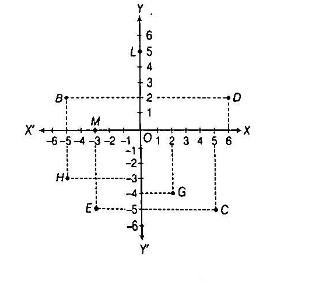Home/Class 9/Maths/

See the figure and find the following:(i) The coordinate of the point B
(ii) The coordinate of the point c
(iii) The point identified by  the coordinates? $$\left(-3,-5\right)$$
(iv) point identified by the coordinates $$\left(2,-4\right)$$
(v) The abscissa of the point D
(vi) The ordinate of the point H
(vii) The coordinates of the point L
(viii) The coordinates of the point MSpeed
00:00
02:34## QuestionMathsClass 9

See the figure and find the following:(i) The coordinate of the point B
(ii) The coordinate of the point c
(iii) The point identified by  the coordinates? $$\left(-3,-5\right)$$
(iv) point identified by the coordinates $$\left(2,-4\right)$$
(v) The abscissa of the point D
(vi) The ordinate of the point H
(vii) The coordinates of the point L
(viii) The coordinates of the point M

(i) Point B is at a distance of 5 units from the Y-axis along the negative direction and 2 units from the X-axis along positive direction. Hence the coordinates of the point B are $$(-5,2)$$
(ii) Point C is at a distance of 5 units from the Y-axis along the positive direction and 5 units from the X-axis along negative direction. Hence the coordinates of the point B are $$(5,-5)$$
(iii) Point, which has distance 3 units from the Y-axis along negative X-axis and 5 units from the X-axis along negative Y-axis is E.
(iv) Point, which has distance 2 units from the Y-axis along positive X-axis and 4 units from the X-axis along negative Y-axis is G.
(v) Point D at a distance of 6 units from the Y-axis along positive X-axis. Therefore its abscissa is 6.
(vi) Point H at a distance of 3 units from the X-axis along negative Y-axis.
Therefore, its ordinates is $$-3$$
(vii) Point L is lie on the Y-axis and which is 5 units from the X-axis along the positive Y-axis. Hence, the coordinates of L is $$\left(0,5\right).$$
(viii) Point M is lie on the X-axis and which is 3 units from the Y-axis along the negative X-axis. Hence, the coordinates of L is $$\left(-3,0\right).$$